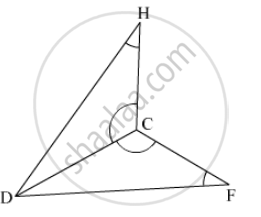SSC (English Medium) Class 8Maharashtra State Board
Share

# In Each Pair of Triangles Given Below, Parts Shown by Identical Marks Are Congruent. State the Test and the One to One Correspondence of Vertices by Which Triangles in Each Pair Are . - SSC (English Medium) Class 8 - Mathematics

ConceptCongruence of Triangles

#### Question

In the triangle given below, parts shown by identical marks are congruent. State the test and the one to one correspondence of vertices by which triangles in each pair are congruent and remaining congruent parts.#### SolutionIn ∆DHC and ∆DFC,
∠DCH ≅ ∠DCF (given)
∠DHC ≅ ∠DFC (given)
side DC is common
∴ By AAS test, ∆DHC ≅ ∆DFC.
∴ side DH ≅ side DF,
side HC ≅ side FC,
∠HDC ≅ ∠FDC.

Is there an error in this question or solution?

#### APPEARS IN

Balbharati Solution for Balbharati Class 8 Mathematics (2019 to Current)
Chapter 13: Congruence of triangles
Practice Set 13.2 | Q: 1.3 | Page no. 87
Solution In Each Pair of Triangles Given Below, Parts Shown by Identical Marks Are Congruent. State the Test and the One to One Correspondence of Vertices by Which Triangles in Each Pair Are . Concept: Congruence of Triangles.
S